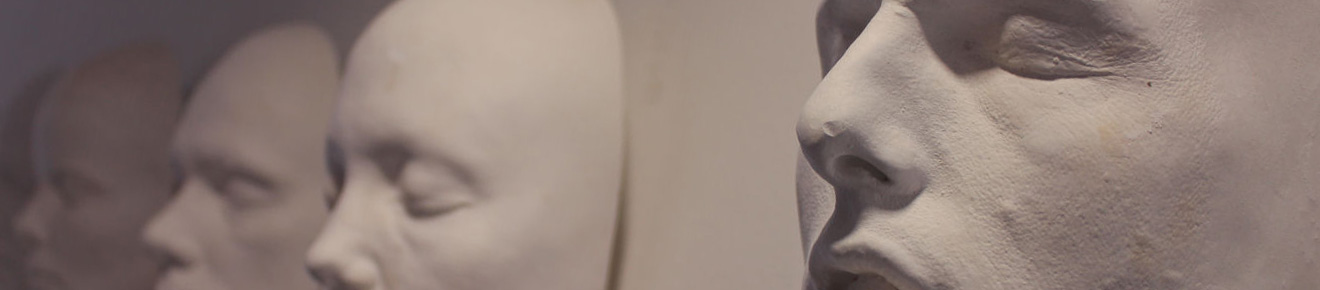# Inverse Modelling of Material Parameters for Rubber-like material: Create a New Methodology of Predicting the Material Parameters using Indentation Bending Test

Seng, JAC (2015) Inverse Modelling of Material Parameters for Rubber-like material: Create a New Methodology of Predicting the Material Parameters using Indentation Bending Test. Doctoral thesis, Liverpool John Moores University.Text 158261_Inverse Modelling of Material Parameters for Rubber.pdf - Published Version Download (4MB)

## Abstract

Rubber-like materials and thin membrane materials have been widely used in industries, such as engineering fields and biomedical fields. The mechanics of membranes and material parameters identification is an important research area. In this work, different inverse FE modelling approaches to extract material parameters (Ogden model) has been assessed supported by several newly developed programs in producing or analysing the data. The effects of new material properties (e.g. negative Poisson’s ratio) are also studied.

In this work, the use of inverse FE modelling and indentation bending test for material property identification has been systematically studied. A parametric FE model has been developed and validated simulating the indentation bending tests of thin latex rubber samples made in the laboratory. Rubber samples were tested in standard uniaxial tensile, planar tests, the hyperelastic material parameters are determined and used as the target for an inverse materials parameter identification program. An ABAQUS add-on program has been developed to automatically update the material parameters and extract the force-displacement data. Simulation spaces over a wide range of material parameters have been developed, which successfully provided the numerical data for the inverse approach for material property prediction. Different curve analysis approaches in representing the force-displacement curve have been proposed including (1) using ratio of P/h3 for the low load region, (2) using the effective slope at higher load and (3) using the second order polynomial curve fitting parameters. The result shows that use of these curve fitting parameters could effectively simplify the inverse FE modelling process and allow the use of surface plot equations to establish a mathematical relationship between curve coefficients and material parameters. These relationships could effectively open up the possibility of improving the uniqueness of inversely predicted material property sets by combining either data from different testing conditions or different curve data from the same test.

As an important part of the work, several new programs have been developed to process and analyse the data. A MatLab program has been developed to determine the surface equation between the key curve coefficients and the material parameters based on FE data with an Ogden model. Work based on the data from a single indenter test shows that there are multiple material property sets that could produce identical force-displacement data. This confirms that the results are not unique. Several approaches have been evaluated by combining the surface equation for different curve parameters or testing conditions (i.e. sample sizes) to improve the robustness of the inverse prediction. A program has been developed and implemented by the inverse FE modelling which allows systematic studies with different approaches including dual chamber size, thickness or combining a two curve parameter approach. The results demonstrate combining different curve fitting approaches with a smaller chamber size is more effective than using the dual chamber size approach. Relaxation indentation bending test have been performed and different inverse FE program has been comparatively applied to estimate the viscoelastic parameters from the test data. One program is based on full objective function approach; the other is a two staged interactive searching approach. This program has been evaluated in both training data using numerical data as the target and relaxation test data of the natural latex rubber sample.

The deformation of circular elastic membranes with a clamped edge under point loading or finite contact conditions is systematically studied incorporating auxeticity behaviour (Negative Poisson’s ratio). The effect of Poisson’s ratio on the deformation of the material is established. The feasibility and limitation of an analytical solution is assessed. The work shows that the P/h3 relationship is applicable to describe the force displacement data over the membrane domain for both point loading and finite contact conditions. It is shown that negative Poisson’s ratio has shown clear influence on the membrane deformation domain; the resistance force of a membrane with a negative Poisson’s ratio is relatively lower, which could be beneficial for application such as sensors, as the material will be more sensitive to load change. This work has highlighted some important characteristics of membranes with negative Poisson’s ratio, further work is required to quantify these effects with consideration of relative dimensions between sample thickness and chamber size.

Item Type: Thesis (Doctoral) Indentation bending test T Technology > TA Engineering (General). Civil engineering (General) Maritime & Mechanical Engineering (merged with Engineering 10 Aug 20) 04 Nov 2016 12:01 03 Sep 2021 23:27 10.24377/LJMU.t.00004514 Ren, J, Allanson, D and Li, R https://researchonline.ljmu.ac.uk/id/eprint/4514View Item